Areas of Science Sports Science Pure Mathematics Difficulty Time Required Short (2-5 days) Prerequisites You should have a familiarity with basic algebra. Material Availability Readily available Cost Very Low (under \$20) Safety No issues

## Abstract

If you've ever played or watched basketball, you might already know that your chances of successfully banking a shot on the backboard are higher in certain positions on the basketball court, even when keeping the distance from the hoop the same. Ever wondered what would account for this? Do you think you could actually explain this using geometry? This science project will put your knowledge of geometry and algebra to good use. You will calculate and quantify how much more difficult it is to score from different positions. You will also build a scale model to test your calculations. Will these results line up with the on-court experience?

## Objective

Use algebra and geometry to calculate the relative probability of making a successful bank shot from different positions on the court, keeping the distance to the hoop constant. Then test if you can reproduce your predictions with a scale model.

## Share your story with Science Buddies!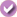Yes, I Did This Project! Please log in (or create a free account) to let us know how things went.

## Credits

Sabine De Brabandere, PhD, Science Buddies

General citation information is provided here. Be sure to check the formatting, including capitalization, for the method you are using and update your citation, as needed.

### APA Style

Last edit date: 2020-01-12

## Introduction

People say playing sports helps improve academic results. Would academic analysis of the game also improve your performance on the court? Test it out with this science project. Have a close look at the geometry of shooting a basket using the backboard. Would geometric analysis indicate that shooting from the side of the hoop—as shown in Figure 1—is easier, more difficult, or equally difficult than shooting from a more central position? Could geometric analysis help you make better decisions on the court?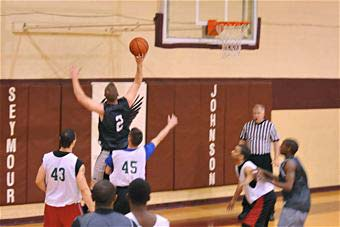Figure 1. Making a shot from a sideways position relative to the hoop.

A basketball shot at the hoop is a great example of projectile motion, or the motion of an object (in this case a ball) that moves through the air. The science of projectile motion lets you predict the projectile's (here a ball's) trajectory, or the exact path the projectile (or ball) will take.

In real basketball, the ball follows a complicated three-dimensional trajectory as it moves through the air, bounces off the backboard, and goes into the net. However, it is an example of a compound motion, combining a vertical and a horizontal motion. Both these motions are independent of each other, and thus, can be studied separately. In this science project, you will study the horizontal movement of the basketball, which reduces the three-dimensional motion to two dimensions. This "bird's-eye view" of the ball's movement will result in easier analysis for your science project.

Are you surprised that the ball's vertical and horizontal motions happen independently?

You can experiment with this type of compound motion by letting identical objects (like two coins) fall to the ground using different paths (e.g. one coin launched off sideways from a table, while the second one is nudged right off the edge and falls straight down from the table). See when both objects land. If vertical movement (falling to the ground) happens independent of the horizontal movement, both coins should touch the ground at the same time, even though the first object took a longer path.

Your goal is to determine the relative difficulty to shoot the basket using the backboard from different positions on the court. Imagine looking at the court from a bird's-eye (top-down) viewpoint. Now imagine a player is shooting a ball. What would you see? Can you visualize the trajectory of the ball being shot, bouncing off the backboard, and landing in the basket? Do you imagine a trajectory as shown in Figure 2?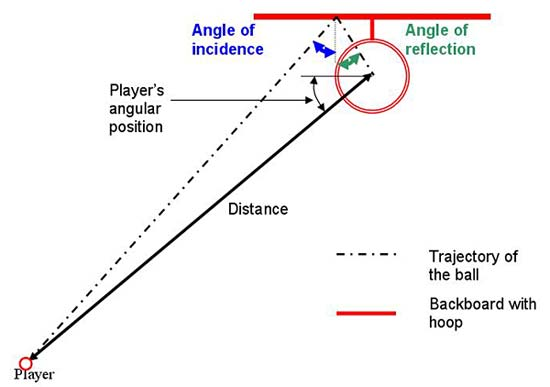Figure 2. Top-down view of the trajectory of a banked shot. This figure also shows the angle of incidence being equal to the angle of reflection, as it is assumed the ball has no spin.

Figure 2 also introduces the angle of incidence and the angle of reflection for a projectile. The angle of incidence is the angle between the path of the ball as it approaches a surface (here the backboard) and the line perpendicular to the surface (a line perpendicular to a surface is also called normal to the surface or the surface normal ) . The angle of reflection represents the angle between the path of the ball as it bounces back from a surface and the line perpendicular to the surface. In this science project, you will assume the ball is thrown without spin. In this case, projectile motion predicts the ball will bounce back under the same angle, or in other words, the angle of incidence equals the angle of reflection.

In the first part of your science project, you will use algebra and geometry to study one aspect of the shot and determine its impact on relative difficulty of making a shot from different positions that are the same distance from the hoop. Here is a short review of some properties of triangles that will be used in the calculations.

The Pythagorean theorem is probably the first thing that comes to mind when thinking of triangles. It is valid in right triangles and states that the square of the hypotenuse (the length of the side opposite the right [90°] angle) equals the sum of the squares of the length of the other two sides.

Do you also remember the special properties of the 30-60-90 and the 45-45-90 triangles?

A 30-60-90 triangle is a triangle with 30°, 60° and 90° angles, as shown in Figure 3. One side of this triangle has a length exactly half of the length of the hypotenuse. Can you use the Pythagorean theorem to verify that the length of the other side is $\sqrt{3}/2$ times the length of the hypotenuse?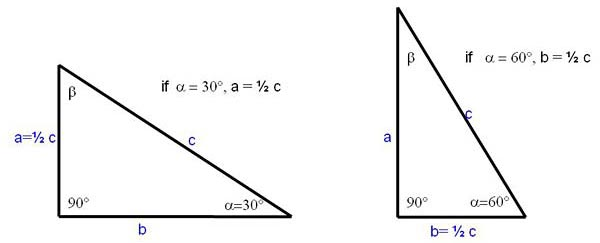Figure 3. Representation of the 30-60-90 triangle, for which the length of one side is half the length of the hypotenuse. In this figure, the lengths a, b, and c represent the length of each side of the triangle, while the Greek letters α and β represent the angles.

A 45-45-90 triangle is special because two sides of this right triangle have equal lengths. Can you use the Pythagorean theorem to verify this length is exactly $\sqrt{2}/2$ times the length of the hypotenuse?

You will also need to know the properties of similar triangles. Similar triangles are triangles that have identical angles, but might differ in size. Figure 4 shows two similar triangles. Note the corresponding sides of similar triangles have lengths in the same ratio. In other words, if a triangle with sides a, b, and c is similar to a triangle with sides A, B, and C, then a/A = b/B= c/C.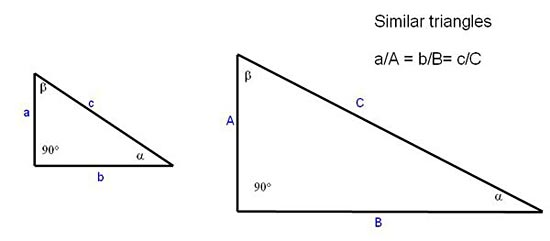Figure 4. Representation of two similar triangles. Note that the ratios of the lengths of corresponding sides of similar triangles are identical.

There is one more mathematical concept to introduce before you can start your calculations. In this science project, you will study one aspect of the shot and calculate how this influences chances of scoring when the player is placed at different locations on the court. Scientists use relative probability to express how much more or less likely something is expected to happen compared to a chosen baseline. A relative probability higher than 1 indicates it is more probable, or easier, to successfully shoot a banked shot from that position than from the baseline position. A relative probability lower than 1 indicates it is less probable, or more difficult, to successfully shoot a banked shot from that position than it is from the baseline position.

Now, time to get started! Make sure you keep your science project in mind the next time you are deciding whether or not to use the backboard to take a shot!

## Terms and Concepts

• Projectile motion
• Trajectory
• Compound motion
• Independent
• Angle of incidence
• Angle of reflection
• Pythagorean theorem
• Right triangles
• Hypotenuse
• 30-60-90 triangle
• 45-45-90 triangle
• Similar triangles
• Relative probability

### Questions

• What property allows analysis of the projectile motion in the horizontal plane and the vertical direction separately?
• How does the trajectory of a basketball shot look in the horizontal plane?
• What is the angle of incidence and the angle of reflection for a ball hitting an object and how are they related, assuming the ball has no spin?
• What does relative probabilities of 200% or 50% indicate?
• How are the lengths of the three sides of a right triangle related?
• Do you expect any position on the court to have a higher chance of resulting in a score using the backboard when analyzing the geometry of the situation?
• Do you expect any players placed on the court at equal distance from the center of the basket to have a better chance of scoring using the backboard in the real game?

## News Feed on This Topic, ,
Note: A computerized matching algorithm suggests the above articles. It's not as smart as you are, and it may occasionally give humorous, ridiculous, or even annoying results! Learn more about the News Feed

## Materials and Equipment

• Test ball, approximately 4.5 cm diameter. A mini sports squeeze ball like the mini sports foam balls from Amazon.com work well.
• Note: You will need to scale the dimensions of the cardboard tube and the number of poster boards up or down to accommodate a smaller or larger ball.
• Books (2), identical thickness
• Flat wall making a 90° angle with a flat floor
• Ruler, metric
• Poster boards (2), 22 x 28 inch; flexible or hard poster board will both work.
• Thick markers, black and red
• Compass
• Protractor
• Sheet of paper
• Tape that does not damage the wall
• Scissors
• Cardboard roll (50 cm long if you work with a 4.5 cm ball); the inner tube of packing paper works well. Note : Choose something that comes on a sturdy cardboard tube, not on a flimsy wrapping paper tube.)
• Cutting board
• Toilet paper roll
• Utility knife or craft knife
• Highlighter
• Lab Notebook

Disclaimer: Science Buddies participates in affiliate programs with Home Science Tools, Amazon.com, Carolina Biological, and Jameco Electronics. Proceeds from the affiliate programs help support Science Buddies, a 501(c)(3) public charity, and keep our resources free for everyone. Our top priority is student learning. If you have any comments (positive or negative) related to purchases you've made for science projects from recommendations on our site, please let us know. Write to us at scibuddy@sciencebuddies.org.

.PDF date: 2020-01-22

## Experimental Procedure

### Defining the Variables

For this science project, you will position your player at a fixed distance from the middle of the basket. Three meters (m) will be used as the example in this procedure. Feel free to replace this distance by your own preferred distance. It is important to stick to one distance throughout the project, though, as you want to study the influence of the player's angular position. Also note that metric units are used, as this is the convention for science projects.

Once you have specified the distance, the position of the player is defined by the angle that the line from the player through the middle of the basket makes with a line parallel to the backboard, as shown in Figure 5.Figure 5. Players are positioned at a constant distance from the center of the basket and varying angular positions. The 90° position is chosen as baseline, which means the probabilities will be calculated relative to the 90° position.

The 90° position is chosen as baseline, which means you will calculate how much more or less difficult it is to make the shot from the 30°, 45°, and 60° positions with respect to the 90° position.

### Calculating the Chance to Score

Depending on the player's angular position, the player will have to aim the ball at a different location on the backboard for the ball to end up in the basket, as shown in Figure 6.Figure 6. Players at different locations need to aim the ball at a different spot on the backboard to make the ball bounce into the basket.

The player always aims to get the ball in the middle of the basket. Fortunately, there is some wiggle room. As shown in Figure 7, the ball can bounce off of a slightly different spot on the backboard and still bounce into the basket.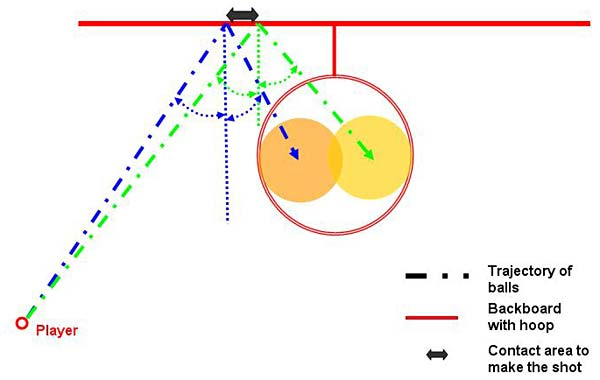Figure 7. A player placed at a specific position can throw a ball to a slightly different spot on the backboard and still make the shot. The contact area on the backboard that will result in a shot can be used as an indicator of how difficult it is to shoot from that position.

How much wiggle room a player has is one indicator of how difficult it is to make the shot. You can calculate relative probabilities by comparing the length of this "wiggle room" (the length of the area on the backboard that a player can hit and still make the shot) for different player positions. In this approach, you calculate the relative sizes of the areas at which a player can choose to aim as explained later in the procedure. This works if you assume that the player can aim the ball perfectly every time. Another approach is to calculate the angular wiggle room the player has when aiming the ball (for example, plus or minus two degrees), assuming the player knows exactly which spot on the backboard to aim at. The calculations are slightly different in this case, as explained in the Variations section. In the real game, the relative probability is a combination of both of these and other aspects.

Some general notes before you start the calculations:

• Although in sports the distances are often indicated in inches and yards, calculations will be done in the metric system (centimeters and meters). Be sure to make appropriate conversions where needed.
• All figures indicate the angle defining the position of the player as β. The first time you go through these calculations, fill in the angle 30°. At later iterations, you will do the calculations for the 45°, the 60°, and the 90° positions. Fill in the appropriate number for β each time you go through the calculations.
• At any step in your calculations, remember to verify if the obtained distances make sense. As an example, a ball hitting the backboard at 10 m away from the center of the backboard does not seem correct. Frequent checks will help you catch mistakes early on.
1. Copy the table in your lab notebook; it will allow you to systematically keep track of your calculations as you go through them for different angular positions.
1. Note: If you are able to work symbolically until you arrive at an expression for X1 as a function of the other variables (β, Xtot, Ytot, ...)- please do set up the equation and plug in the numbers at the end. If this is difficult for you, plug in numbers at each step.
 Player's positiondefined by angle β 30° 45° 60° 90° Ball lands central Xtot (m) Ytot (m) Y2 (m) Y1 (m) X2 (m) X1 (m) Ball lands left Xtot (m) Ytot (m) Y2 (m) Y1 (m) X2 (m) X1 (m) Ball lands right Xtot (m) Ytot (m) Y2 (m) Y1 (m) X2 (m) X1 (m) Length contact line (m) Relative probability 1

Table 1. Table in which to note down the intermediate and end results of your calculations.
1. Make a drawing similar to the one shown in Figure 8. Concentrate on the yellow triangle.
1. Calculate the length of all sides of the yellow right triangle. Note that you know angle β and the length of the hypotenuse.
2. Add the appropriate distances, if any, to obtain the length of Xtot (the distance in the X direction from the player's position to the middle of the ball as it enters the hoop) and Ytot (the distance in the Y direction from the player's position to the backboard) indicated in black in the figure. Note that you might have to convert inches into meters.
3. Note your results down in your lab notebook in the "Ball lands central" rows in your table like Table 1.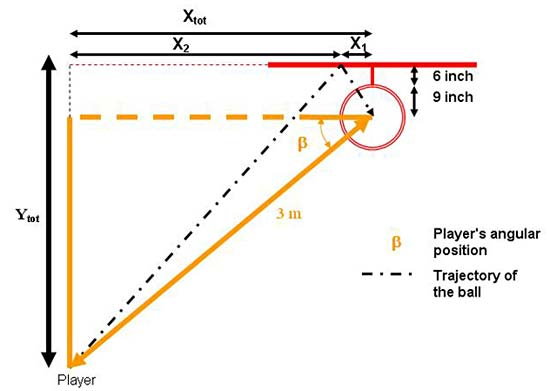Figure 8. A drawing of the setup helps identify the geometry and translate the problem into a mathematical equation.
1. Make a drawing similar to the one in Figure 9. You want to find X1, as this will indicate the distance from the center line where the ball needs to hit the backboard and bounce back into the basket. Note that the drawing is made to reflect that the ball bounces back into the center of the basket. The center line refers to the line perpendicular to the backboard going trough the center of the basket.
1. Concentrate on green (with sides X2, Y2, Z2) and blue (with sides X1, Y1, Z1) triangles. Since the angle of incidence is equal to the angle of reflection, these two triangles are similar. Use this information to write down an equation between Y1, Y2, X1, X2.
2. As you can see from the Figure 9, Y2 and Ytot are identical. You also know Y1, and you are trying to find X1. You still need to identify X2.
3. Since you know Xtot from step 2, find an equation that relates X 1, X2, and Xtot.
4. Use the equation obtained in step 3.c. to express X2 as a function of X1 and Xtot.
5. Substitute your expression for X2 in terms of X1 and Xtot in the equation you formed in step 3.a.
6. Reorganize your expressions so you get X1 as a function Ytot, Y1, and Xtot.
7. If you did not do it yet, plug the values for Ytot, Y1, and Xtot into your expression from step 3.f., and solve for X1. Record your result in your data table next to the variable X1 (m) - ball lands central.
8. Do a reality check; is the spot indicated by your distance still within the backboard?Figure 9. The mathematical properties of similar triangles (drawn in green and blue in this figure) are used to solve the problem.
1. Repeat step 3. When necessary, change your procedure to account for the situation where the ball barely makes it through the left edge of the basket, as shown in Figure 10 (light yellow ball).
1. Start by making a new drawing or by adding the new situation to your previous drawing in a different color.
2. Identify which, if any of the variables Ytot, Y1, Y1, X2, and Xtot are unchanged. Record these in your data table.
3. For the variables that do change, do you know by how much they will change? For example, Xtot will be less or more as the ball will enter to the left or to the right of the central position. Can you identify how much less, knowing a basketball has a diameter of 9.4 inches and the radius of the basket is 9 inches?
4. Can you see if the relationships between the variables you established in step 3.a. and 3.b. still hold? If so, you can use the relationship established in step 3.f. with the new values of Ytot, Y1, and Xtot to calculate the distance X1 (m) - ball lands left. This is the farthest point to the left for the angle β that will still result in the ball bouncing into the basket.
5. Do a reality check; is the spot indicated by your distance still within the backboard?Figure 10. Figure showing the situation where the ball barely makes it through the basket to the right (light blue) or to the left (light yellow) of the central position.
1. Repeat step 4 considering the situation where the ball barely makes it through the basket to the right of the central position, as shown in Figure 10 (light blue ball). This calculation will yield distance X1 (m), ball lands right. This is the farthest point to the right for this angle β that will still result in the ball bouncing into the basket.
2. To calculate the length of the area on the backboard that will result in the ball bouncing into the basket, subtract distance [X1 (m) - ball lands right] from distance [X1 (m) - ball lands left]. Note your results down in your data table. This completes your calculations for angle 30°. Once you have done the calculations for all the angles in the following steps, you can calculate the relative probability.
3. Repeat steps 2–6 again for the following positions: 45° and 60°.
4. Repeat steps 2–6 again for the 90° position. Note that this position looks slightly different, as indicated in Figure 11. Verify that the equations you identified are still valid for this configuration.Three different trajectories of a basketball bouncing off of the backboard and into the basket when shot from a position perpendicular to the backboard. A basketball can be bounced slightly to the left or right of center and still make it into the basket due to the size of the basket being larger than the ball.

Figure 11. Figure showing the configuration for the 90° position for the ball entering in the center (orange), to the right (light blue), or to the left (light pink) of the central position.
1. Now that you have obtained the lengths that make the shot, you are ready to calculate the relative probability.
1. Divide the obtained length for each position by the length for the 90° position. Note the 90° position should have a "1" as relative probability, as this position was chosen as the baseline.
2. Do your results confirm or contradict what you expected? Does it seem logical in retrospect?

### Testing Your Calculations with a Scale Model

Now that you have calculated the relative probability using geometry, you can test if you can reproduce these numbers using a scale model. How to build the scale model and take data is explained in the procedure of the science Project Idea Basketball: Will You Bank the Shot?

Put your players in your scale model at the same positions as you did for your calculations: a 3 m distance from the center of the hoop (scaled down to the distance in your model) and at 30°, 45°, 60°, and 90° angles.

Use the data from your scale model trails to calculate the length of the area on the backboard that bounces the ball back into the basket and the relative probabilities.

### Comparing and Analyzing Your Results

This section will provide you with some information about how to analyze your results.

1. Compare the results obtained from your scale model with the results obtained from your calculations:
1. For each angular position, compare the length of the contact line that made the shot.
2. Compare for each angular position the obtained relative probability.
2. If you see any differences between your scale model results and your calculated results, try to answer the following questions:
1. Can you explain what causes these differences?
2. Is the result of your scale model more accurate than, less accurate than, or the same as the results of your calculations?
3. Can you think of ways to improve your calculations or your scale model?
3. Make scatter plots of your data. Where possible, plot both your calculated results and test model results on the same graph, using color coding to differentiate between the calculated and scale model results. Here are some suggestions:
1. Plot your relative probability (on the y-axis) versus the player's position (angle).
2. Plot the length of the area that results in a score (on the y-axis) versus the player's position (angle).
3. Plot both the minimum distance from the central line and the maximum distance from the central line on the y-axis versus the player's angular position (angle) on the x-axis.
4. Do you see a trend in your plots or your data? What could you conclude? Does one position result in more scores using the backboard than the other?
5. Are your findings in alignment with what you expected?
1. If you play basketball, did you have a similar experience on the court?
2. If you can find "shot charts" for real basketball players indicating the success rate of banked shots for different positions on the court, compare them with your findings.
3. Can you explain any differences identified in step 5.a. or 5.b.? What other factors (geometric and non-geometric) might influence your chance to score. As an example, would aiming be more accurate at large or small angles?
4. You constrained yourself to two dimensions for this science project; would adding the vertical motion change your calculations?
.

## If you like this project, you might enjoy exploring these related careers:

### Mathematician

Mathematicians are part of an ancient tradition of searching for patterns, conjecturing, and figuring out truths based on rigorous deduction. Some mathematicians focus on purely theoretical problems, with no obvious or immediate applications, except to advance our understanding of mathematics, while others focus on applied mathematics, where they try to solve problems in economics, business, science, physics, or engineering. Read more

### Athletic Trainer

Sports injuries can be painful and debilitating. Athletic trainers help athletes, and other physically active people, avoid such injuries, while also working to improve their strength and conditioning. Should a sports injury occur, athletic trainers help to evaluate the injury, determine the treatment needed, and design a fitness regime to rehabilitate the athlete so he or she is ready to go out and compete again. Read more

### Statistician

Statisticians use the power of math and probability theory to answer questions that affect the lives of millions of people. They tell educators which teaching method works best, tell policy-makers what levels of pesticides are acceptable in fresh fruit, tell doctors which treatment works best, and tell builders which type of paint is the most durable. They are employed in virtually every type of industry imaginable, from engineering, manufacturing, and medicine to animal science, food production, transportation, and education. Everybody needs a statistician! Read more

### Physicist

Physicists have a big goal in mind—to understand the nature of the entire universe and everything in it! To reach that goal, they observe and measure natural events seen on Earth and in the universe, and then develop theories, using mathematics, to explain why those phenomena occur. Physicists take on the challenge of explaining events that happen on the grandest scale imaginable to those that happen at the level of the smallest atomic particles. Their theories are then applied to human-scale projects to bring people new technologies, like computers, lasers, and fusion energy. Read more

## Variations

• In this science project, you changed the position of the player by changing the angle and keeping the distance to the center of the hoop constant. Try the project again, this time keeping the angle constant and analyzing the influence of changing the distance to the hoop.
• If you have some basic trigonometry knowledge, you can find a symbolic expression for the relative probability as a function of the player's angular position. Then plot this function, with relative probability on the y-axis and the player's angular position on the x-axis. This will give you information about how the relative probability changes with changing angular position, taking all positions (all angles) into account.
• Ask some of your basketball-playing friends to test their success rates from the analyzed positions, using the backboard. Compare the average of these real-life results with the results obtained in your calculations and/or your test situation. Analyze what might account for the differences between your calculations, your scale model, and shooting on an actual court.
• The calculations in this science project assume that the player can throw the ball with perfect aim every time. In reality, there may be some error when the player throws the ball, resulting in the ball going a few degrees to the left or to the right, as shown in Figure 12. Can you calculate the relative probabilities using this angular "wiggle room" instead of the linear wiggle room on the backboard? How do the two methods compare; do they give the same results, or are they different?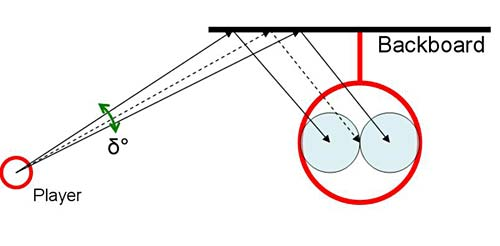Three different trajectories of a basketball bouncing off of the backboard and into the basket when shot from the left side of the basket. A basketball can be bounced slightly to the left or right of center and still make it into the basket due to the size of the basket being larger than the ball.

Figure 12. You can also calculate the relative probability to successfully bank a shot by comparing the angular wiggle room for different positions on the court. The player displayed in this drawing has wiggle room of δ degrees.
• Use geometry and algebra to analyze in which direction you should throw the ball for a running player to catch it.
• Look up "shot charts" for real basketball players (for example, do an online image search for "NBA shot chart") and compare your data to these shot charts. Does data from real basketball games match the trends you saw in your experiment, or not? What other factors do you think could influence a real game that were not present in your experiment?

## Share your story with Science Buddies!Yes, I Did This Project! Please log in (or create a free account) to let us know how things went.

The Ask an Expert Forum is intended to be a place where students can go to find answers to science questions that they have been unable to find using other resources. If you have specific questions about your science fair project or science fair, our team of volunteer scientists can help. Our Experts won't do the work for you, but they will make suggestions, offer guidance, and help you troubleshoot.